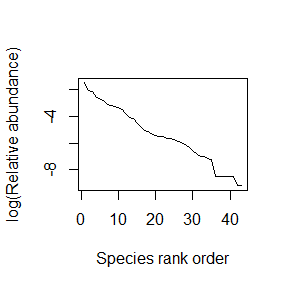## Online Box 7: R code to simulate a neutral model in one large community with speciation: Figure 6.11

This code produces only one of the scenarios in Figure 6.11. The parameter nu needs to be altered to generate the other scenarios.

WARNING 1: this code takes a while to run (~20 minutes or more)

WARNING 2: More than half the code is post-simulation calculations in order to generate graphics. The end result (a relative abundance distribution) is needed for the code in Online Box 8

## specify parameters, initial conditions, and output vector
num.years <- 10000
J <- 10000
sp <- 1 # a counter to keep track of added species
COM <- vector(length = J); COM[] <- sp

nu <- 5e-04 # speciation rate

year <- 2

COM.out <- matrix(nrow = (num.years/100+1), ncol = J); COM.out[1,] <- COM

## run simulation
for (i in 1:(J*(num.years))) {

## speciation (replace dead individual with new species)
if (runif(1) < nu) {
sp <- sp + 1
} else {

## not speciation (replace dead individual from community)
}

## record data every 100 years
if (i %% (J*100) == 0) {
COM.out[year,] <- COM
year <- year+ 1
} }

################################
# Calculations to allow plotting relative abundance distributions

## First calculate the number of species (SR) at each time

SR.out <- vector(length = dim(COM.out))

for (t in 1:dim(COM.out)) {
COM.hist <- hist(COM.out[t,],c(1:max(COM.out[t,])),plot=FALSE)
abund.vec <- COM.hist$counts[COM.hist$counts>0]
SR.out[t] <- sum(abund.vec>0)
}

## Calculate species abundances
abund.out <- matrix(nrow = (num.years/100+1), ncol = max(SR.out))
abund.out[] <- 0

for (t in 1:dim(COM.out)) {
COM.hist <- hist(COM.out[t,],c(1:max(COM.out[t,])),plot=FALSE)
abund.vec <- COM.hist$counts[COM.hist$counts>0]
abund.out[t,1:SR.out[t]] <- abund.vec
}

abund.out <- abund.out/J

## The final rank-order of relative abundances

## Write the final relative abundance distribution to a file for use in Online Box 8 (island biogeography model)

## graph the results
xlab="Species rank order", ylab="log(Relative abundance)")### Description of the code.

1. num.years, J, and COM are defined as in previous Boxes. nu is the speciation rate. With probability nu, the dead individual will be replaced by a species new to the metacommunity. Since we will have an undetermined number of species, sp will keep track of species identities. The first species is species 1, and each new species will be numbered in sequence.
num.years <- 100
J <- 100
sp <- 1 # a counter to keep track of added species
COM <- vector(length = J); COM[] <- sp

nu <- 1e-04 # speciation rate
1. With many different species, we can no longer just keep track of the frequency of species 1. ncol = J because we will keep track of the identity of each individual in each year of recorded data. In order to reduce the duration of the simulation, we will record data only every 100 years, which is why nrow = (num.years/100+1)
COM.out <- matrix(nrow = (num.years/100+1), ncol = J); COM.out[1,] <- COM 
1. Since we don’t have just one line in the simulation where COM can be updated, we select an individual to die before figuring out if it will be replaced by a speciation even or by a local reproducer.
  dead.indiv <- ceiling(J*runif(1))
1. Here’s where speciation happens. If the new individual is produced by speciation (with probaiblity nu), we update the sp counter, keeping track of how many species have entered the community.
  if (runif(1) < nu) {
sp <- sp + 1
} else {
1. Stop and record data every 100 years (after J*100 deaths) instead of every year (after J deaths) as in previous Boxes).
  if (i %% (J*100) == 0) {
COM.out[year,] <- COM
year <- year+ 1
} } 
1. We need to calculate the number of species present (Species Richness) at each time step (when data were recorded) in order to avoid outputting zero abundances later. dim(COM.OUT) returns the first dimension (number of rows) of COM.OUT, which is the number of time steps at which we recorded data. The hist (histogram) function returns, in the $counts object, a vector of abundances of each species in the community, most of which will be zero (because most species entering via speciation go quickly extinct). We specify plot=FALSE so that R doesn’t produce a histogram graph each time. abund.vec will contain only the non-zero elements, and abund.vec>0 returns a vector with a ‘1’ for each species with non-zero abundance, the sum of which is species richness. SR.out <- vector(length = dim(COM.out)) for (t in 1:dim(COM.out)) { COM.hist <- hist(COM.out[t,],c(1:max(COM.out[t,])),plot=FALSE) abund.vec <- COM.hist$counts[COM.hist$counts>0] SR.out[t] <- sum(abund.vec>0) } 1. Using the same functions as in point 6 above, we calculate a matrix of species relative abundances at teach time point. This is done separately from (and after) the species richness calculations because we don’t need an abundance matrix with any more columns than there were species present at at least one of the data collection time points. Many species arise and disappear within a 100-year window of time. Dividing abundances by J returns relative abundances that sum to one. abund.out <- matrix(nrow = (num.years/100+1), ncol = max(SR.out)) abund.out[] <- 0 for (t in 1:dim(COM.out)) { COM.hist <- hist(COM.out[t,],c(1:max(COM.out[t,])),plot=FALSE) abund.vec <- COM.hist$counts[COM.hist\$counts>0]
abund.out[t,1:SR.out[t]] <- abund.vec
}

abund.out <- abund.out/J
1. final.rad is the rank-order relative abundance vector at the final time step. This needs to be saved if we want to use it as the metacommunity relative abundance distribution in Online Box 8. Note that you will need to create/set the directory on your own (i.e., replaced c:/temp/RAD1.csv with whatever directory and filename you want).
final.rad <- sort(abund.out[(num.years/100+1),1:SR.out[(num.years/100+1)]],decreasing=TRUE)

## Write the final relative abundance distribution to a file for use in Online Box 8 (island biogeography model)
write.csv(final.rad, file = "RAD1.csv")